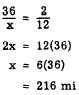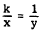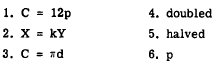Custom SearchWord Problems A knowledge of proportions often provides a quick method of solving word problems. The following problem is a typical example of the types that lend themselves to solution by means of proportion. If an automobile runs 36 mi on 2 gal of gas, how many miles will it run on 12 gal? Comparing miles to miles and gallons to gallons, we have          36:x = 2:12 Rewriting this in fraction form, the solution is as follows:Practice problems, In each of the following problems, first set up a proportion and then solve for the unknown quantity: 1. The ratio of the speed of one aircraft to that of another is 2 to 5. If the slower aircraft has a speed of 300 knots, what is the speed of the faster aircraft? 2. If 6 seamen can empty 2 cargo spaces in 1 day, how many spaces can 150 seamen empty in 1 day? 3. On a map having a scale of 1 in. to 50 mi, how many inches represent 540 mi? Answers: 1. 750 kt  2. 50  3. 10.8 in. VARIATION When two quantities are interdependent, changes in the value of one may have a predictable effect on the value of the other. Variation is the name given to the study of the effects of changes among related quantities. The three types of variation which occur frequently in the study of scientific phenomena are DIRECT, INVERSE, and JOINT. DIRECT VARIATION An example of direct variation is found in the following statement: The perimeter (sum of the lengths of the sides) of a square increases if the length of a side increases. In everyday language, this statement might become: The longer the side, the bigger the square. In mathematical symbols, using p for perimeter and s for the length of the side, the relationship is stated as follows:             p = 4s Since the number 4 is constant, any variations which occur are the results of changes in p and s. Any increase or decrease in the size of s results in a corresponding increase or decrease in the size of p, Thus p varies in the same way (increasing or decreasing) as a. This explains the terminology which is frequently used: p varies directly as s. In general, if a quantity can be expressed in terms of a second quantity multiplied by a constant, it is said to VARY DIRECTLY AS the second quantity. For example if x and y are variables and k is a constant, x varies directly as y, if x = ky. Thus, as y increases x increases, and as y decreases, x decreases. There is a direct effect on x caused by any change in y. The fact that x varies as y is sometimes indicated by x 0~ y, or x - y. However, it is usually written in the form x = ky. The relationship x = ky is equivalent to X/y = k. If one quantity varies directly as a second quantity, the ratio of the first quantity to the second quantity is a constant. Thus, whatever the value of x, where it is divided by y, the result will always be the same value, k. A quantity that varies directly as another quantity is also said to be DIRECTLY PROPORTIONAL to the second quantity. In x = ky, the coefficient of x is 1. The relationship x = ky can be written in proportion form asorNotice that the variables, x and y, appear either in the numerators or in the denominators of the equal ratios. This implies that x and y are directly proportional. The constant, k, is the CONSTANT OF PROPORTIONALITY. Practice problems. Write an equation showing the stated relationship, in each of the following problems: 1. The cost, C of a dozen wrenches varies directly as the price, p, of one wrench. 2. X is directly proportional to Y (use k as the constant of proportionality). 3. The circumference, C, of a circle varies directly as its diameter, d (use p as the constant of proportionality). In the following problems, based on the formula p = 4s, find the appropriate word or symbol to fill the blank. 4. When s is doubled, p will be ________________. 5. When s is halved, p will be _________________. 6. _________________  is directly proportional to s. Answers: